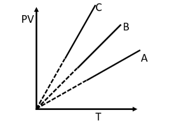Thermometry
Question

# PV versus T graph of equal masses of  ${\mathrm{H}}_{2},\mathrm{He}$ and  ${\mathrm{O}}_{2}$ is shown in the figure.Easy
Solution

## $\begin{array}{l}\mathrm{PV}=\mathrm{nRT}\\ \mathrm{PV}=\frac{\mathrm{m}}{\mathrm{M}}\mathrm{RT}\end{array}$Slope is inversely proportional to molecular weight.

Get Instant Solutions